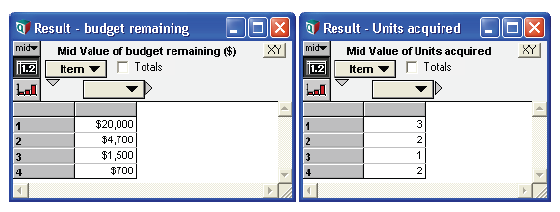# Dynamic on non-Time Indexes

The Dynamic function can be used to express a recurrence along any index, where the value at one value depends on previous values, the same way it is used to express a recurrence along the Time index. For non-Time indexes, you must specify the index explicitly in square brackets following the function name.

In the following example, we are to acquire as much of item 1 as we can afford, but can only purchase an integer number of units. With whatever funds remain, we purchase as many integer units of item 2 as possible, as so on.

Index Item := [1, 2, 3, 4]
Unit_price := Table(Item)(5100, 1600, 800, 250)
Budget := 20K
Num_acquired := Floor(Funds_remaining/Unit_price)
Spent := Num_acquired*Unit_price
Funds_remaining := Dynamic[Item](budget, Self[Item - 1]-Spent[Item - 1])

The three variables, Num_acquired, Spent, and Funds_remaining, form a recurrence over the Item index. Evaluation proceeds starting with the dynamic context Item = 1. When Num_acquired is evaluated in this context, the Unit_price appearing in its definition evaluates to Unit_price[Item = 1], since the use of a variable in a definition refers to its value sliced by the current dynamic context. Evaluation proceeds by evaluating each variable in the loop as Item is incremented and retaining the results for each value of Item: